## Linear Spatial Filters with GNU Octave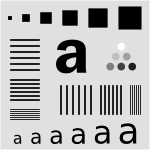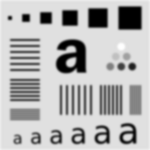I have gotten several e-mails lately about using GNU Octave. One specifically was about blurring images in Octave. In response, I am writing this in-depth post to cover spatial filters, and how to use them in GNU Octave (a free implementation of the Matlab programming language). This should be the sort of information you would find near the beginning of an introductory digital image processing textbook, but written out more simply. In the future, I will probably be writing a post covering non-linear spatial and/or frequency domain filters in Octave.

If you want to follow along in Octave, I strongly recommend that you upgrade to the new Octave 3.0. It is considered stable, but differs significantly from Octave 2.1, which many people may be used to. You will also need to install the image processing package from Octave-Forge. To get help with any Octave function, just type ```help <function>```.

The most common linear spatial image filtering involves convolving a filter mask, sometimes called a convolution kernel, over an image, which is a two-dimensional matrix. In the case of an RGB color image, the image is actually composed of three two-dimensional grayscale images, each representing a single color, where each is convolved with the filter mask separately.

Convolution is sliding a mask over an image. The new value at the mask's position is the sum of the value of each element of the mask multiplied by the value of the image at that position. For an example, let's start with 1-dimensional convolution. Define a mask,

```5 3 2 4 8
```

The 2 is the anchor for the mask. Define an image,

```0 0 1 2 1 0 0
```

As we convolve, the mask will extend beyond the image at the edges. One way to handle this is to pad the image with 0's. We start by placing the mask at the left edge. (zero-padding is underlined)

```Mask:   5 3 2 4 8
Image:  0 0 0 0 1 2 1 0 0
```

The first output value is 8, as every other element of the mask is multiplied by zero.

```Output: 8 x x x x x x
```

Now, slide the mask over by one position,

```Mask:   5 3 2 4 8
Image:  0 0 0 1 2 1 0 0
```

The output here is 20, because 8*2 + 4*1 = 20;

```Output: 8 20 x x x x x
```

If we continue sliding the mask along, the output becomes,

```Output: 8 20 18 11 13 13 5
```

Here is the correlation done in Octave interactively, (`filter2()` is the correlation function).

```octave> filter2([5 3 2 4 8], [0 0 1 2 1 0 0])
ans =

8   20   18   11   13   13    5

```

The same thing happens in two-dimensional convolution, with the mask moving in the vertical direction as well, so that each element in the image is covered.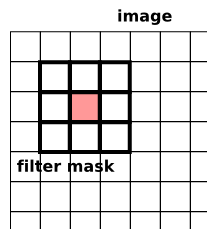Sometimes you will hear this described as correlation (Octave's `filter2`) or convolution (Octave's `conv2`). The only difference between these operations is that in convolution the filter masked is rotated 180 degrees. Whoop-dee-doo. Most of the time your filter is probably symmetrical anyway. So, don't worry much about the difference between these two. Especially in Octave, where rotating a matrix is easy (see `rot90()`).

Now that we know convolution, let's introduce the sample image we will be using. I carefully put this together in Inkscape, which should give us a nice scalable test image. When converting to a raster format, there is a bit of unwanted anti-aliasing going on (couldn't find a way to turn that off), but it is minimal.

Save that image (the PNG file, not the linked SVG file) where you can get to it in Octave. Now, let's load the image into Octave using `imread()`.

```m = imread("image-test.png");
```

The image is a grayscale image, so it has only one layer. The size of `m` should be 300x300. You can check this like so (note the lack of semicolon so we can see the output),

```size(m)
```

You can view the image stored in `m` with `imshow`. It doesn't care about the image dimensions or size, so until you resize the plot window, it will probably be stretched.

```imshow(m);
```

Now, let's make an extremely simple 5x5 filter mask.

```f = ones(5) * 1/25
```

Octave will show us what this matrix looks like.

```f =

0.040000   0.040000   0.040000   0.040000   0.040000
0.040000   0.040000   0.040000   0.040000   0.040000
0.040000   0.040000   0.040000   0.040000   0.040000
0.040000   0.040000   0.040000   0.040000   0.040000
0.040000   0.040000   0.040000   0.040000   0.040000
```

This filter mask is called an averaging filter. It simply averages all the pixels around the image (think about how this works out in the convolution). The effect will be to blur the image. It is important to note here that the sum of the elements is 1 (or 100% if you are thinking of averages). You can check it like so,

```sum(f(:))
```

Now, to convolve the image with the filter mask using `filter2()`.

```ave_m = filter2(f, m);
```

You can view the filtered image again with `imshow()` except that we need to first convert the image matrix to a matrix of 8-bit unsigned integers. It is kind of annoying that we need this, but this is the way it is as of this writing.

```ave_m = uint8(ave_m);
imshow(ave_m);
```

Or, we can save this image to a file using `imwrite()`. Just like with `imshow()`, you will first need to convert the image to `uint8`.

```imwrite("averaged.png", ave_m);
```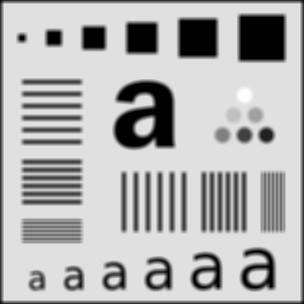There are a few things to notice about this image. First there is a black border around the outside of the filtered image. This is due to the zero-padding (black border) done by `filter2()`. The border of the image had 0's averaged into them. Second, some parts of the blurred image are "noisy". Here are some selected parts at 4x zoom.Notice how the circle, and the "a" seem a little bit boxy? This is due to the shape of our filter. Also notice that the blurring isn't as smooth as it could be. This is because the filter itself isn't very smooth. We'll fix both these problems with a new filter later.

First, here is how we can fix the border problem: we pad the image with itself. Octave provides us three easy ways to do this. The first is replicate padding: the padding outside the image is the same as the nearest border pixel in the image. Circular padding: the padding from from the opposite side of the image, as if it was wrapped. This would be a good choice for a periodic image. Last, and probably the most useful is symmetric: the padding is a mirror reflection of the image itself.

To apply symmetric padding, we use the `padarray()` function. We only want to pad the image by the amount that the mask will "hang off". Let's pad the original image for a 9x9 filter, which will hang off by 4 pixels each way,

```mpad = padarray(m, [4 4], "symmetric");
```

Next, we will replace the averaging filter with a 2D Gaussian distribution. The Gaussian, or normal, distribution has many wonderful and useful properties (as a statistics professor I had once said, anyone who considers themselves to be educated should know about the normal distribution). One property that makes it useful is that if we integrate the Gaussian distribution from minus infinity to infinity, the result is 1. The easiest way to get the curve without having to type in the equation is using `fspecial()`: a special function for creating image filters.

```f_gauss = fspecial("gaussian", 9, 2);
```

This creates a 9x9 Gaussian filter with variance 2. The variance controls the effective size of the filter. Increasing the size of the filter from 9 to 99 will actually have virtually no impact on the final result. It just needs to be large enough to cover the curve. Six times the variance covers over 99% of the curve, so for a variance of 2, a filter of size 7x7 (always make your filters odd in size) is plenty. A larger filter means a longer convolution time. Here is what the 9x9 filter looks like,And to filter with the Gaussian,

```gauss_m = filter2(f_gauss, mpad, "valid";
gauss_m = uint8(guass_m);
```

Notice the extra argument `"valid"`? Since we padded the image before filtering, we don't want this padding to be part of the image result. `filter2()` normally returns an image of the same size as the input image, but we only want the part that didn't undergo (additional) zero-padding. The result is now the same size as the original image, but without the messy border,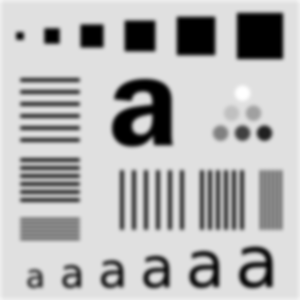Also, compare the result to the average filter above. See how much smoother this image is? If you are interested in blurring an image, you will generally want to go with a Gaussian filter like this.

Now I will let you in on a little shortcut. In Matlab, there is a function called `imfilter` which does the padding and filtering in one step. As of this writing, the Octave-Forge image package doesn't officially include this function, but it is there in the source repository now, meaning that it will probably appear in the next version of that package. I actually wrote my own before I found this one. You can grab the official one here: imfilter.m

With this new function, we can filter with the Gaussian and save like this. Notice the flipping of the first two arguments from `filter2`, as well as the lack of converting to `uint8`.

```gauss_m = imfilter(m, f, "symmetric");
imwrite("gauss.png", gauss_m);
```

`imfilter()` will also handle the 3-layer color images seamlessly. Without it, you would need to run `filter2()` on each layer separately.

So that is just about all there is. `fspecial()` has many more filters available including motion blur, unsharp, and edge detection. For example, the Sobel edge detector,

```octave:25> fspecial("sobel")
ans =

1   2   1
0   0   0
-1  -2  -1
```

It is good at detecting edges in one direction. We can rotate this each way to detect edges all over the image.

```mf = uint8(zeros(size(m)));
for i = 0:3
mf += imfilter(m, rot90(fspecial("sobel"), i));
end
imshow(mf)
```Happy Hacking with Octave!

Have a comment on this article? Start a discussion in my public inbox by sending an email to ~skeeto/public-inbox@lists.sr.ht , or see existing discussions.